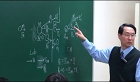### 주메뉴

### 원자력공학개론

• 포항공과대학교
• John C. Lee• 주제분류
공학 >정밀ㆍ에너지 >원자력공학
• 강의학기
2012년 1학기
• 조회수
15,546
•
This course will cover an introduction to nuclear power plants, interaction of radiation with matter, neutron cross sections, diffusion theory, criticality calculations, reactor kinetics, neutron slowing down theory, heat transport and temperature distribution in reactor core, and reactivity feedback.
introduction on new concepts and discussion#### 차시별 강의1Introduction introduction to nuclear power plants 2introduction on new concepts introduction to nuclear physics, cross section, npp accidents, 3introduction on new concepts introduction of mechanisms of nucleus-ceutron interactions 4introduction on new concepts and discussion introduction of two-body collision mechanics and discussion5introduction on new concepts introduction of differential cross secion, scattering kernel, and remarks on cross section6introduction on new concepts introduction of Angular neutron flux and scalar flux7review review on angular and scalar flux, collimated beam8introduction on new concepts introduction on current, parital current, reaction rate, effective cross sections9introduction on new concepts introduction on Maxwell-Boltzman distribution, 1/v-absorber10Introduction introduction on Four-factor formular, derivation of neutron diffusion equation11Class cancelled due to KNS meeting 12Ficks law, one-group diffusion equation introduction on Ficks law, one-group diffusion equation13Introduction and discussion introduction and discussion on B.C. for diffusion equation, solution to diffusion equation, PS#314Introduction on new concept introduction on Albedo, kernel techniques, criticality condition15Holiday 16Introduction on new concepts and Review Introduction on finite diffference solution, Eigenvalue, reactivity and review #1,17Exam 18Introduction on new concepts Introduction on Finite different formulation, Gaussian elimination19Holiday 20Introduction on new concepts introduction on Outer iteration, relative power, flux synthesis, two-group equation21Introduction on new concepts Derivation of point kinetics equation, Laplace transform22Introduction on new concepts introduction on Solution of point kinetics equation, prompt jump, inhour equation23Introduction on new concepts and discussion introduction on transfer function, simulink and discussion on PS#524introduction on new concepts introduction on Slowing down density, collision density for H-medium25Exam 26introduction and review Exam review, resonance integral, T/H analysis of nuclear reactors#### 연관 자료#### 사용자 의견

강의 평가를 위해서는 로그인 해주세요.

#### 이용방법

• 강의 이용시 필요한 프로그램 [바로가기]

※ 강의별로 교수님의 사정에 따라 전체 차시 중 일부 차시만 공개되는 경우가 있으니 양해 부탁드립니다.